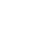# Discount and capitalization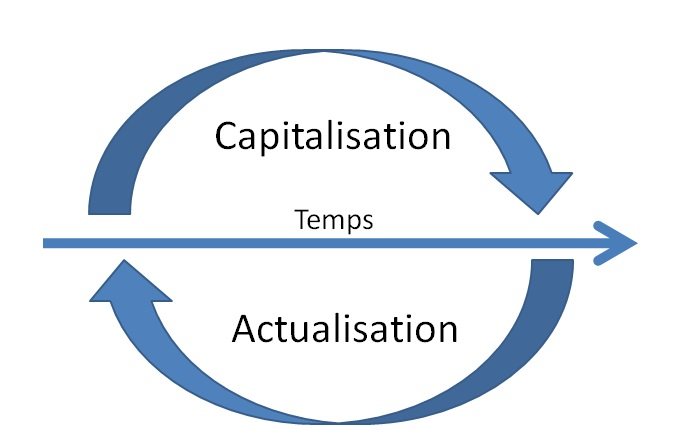Capitalization:

Capitalization comes down to calculating the value of capital after a number of periods since the start of the operation.

Answered the question how I'll money with placed euros X to y percent after Z time

Refresh:

Refresh returns to calculate the value of a capital since its value today in the future.

Answered the question how are now worth X future euros that have been placed to y percent after Z time

We will address the financial calculations allowing to focus on key finance issues.

# What to know before you go any further

Before you begin this first theme, it is necessary to study some knowledge referring to the interest rate used.

## Terms used to refer to the interest rate

-The annual rate

In order to define a rate used during a financial transaction, we use the term annual rate. The latter can be calculated in proportion to the corresponding rate if the calculation time is less than a year.

Example

• Or an annual rate of 6%

Question: What is the monthly rate and the proportional trimestriell rate

The proportional monthly rate: 5% x 1/12 = 0.05

The proportional quarterly rate: 6% x 3 12 = 1.5

Proportional attention does not mean equivalent, having annual 6% or 0.05% monthly is not the same, because having 0.05 monthly allows you to reinvest and ultimately to have a little more than 6% annual.

## Fixed rate or variable rate?

In financial operation, it may be necessary to use two different terms:

• Variable rate: a rate which may be revised at certain deadlines by a clearly determined the market rate.
• Fixed rate: it is a rate that will not change until the end of the financial operation.

## Nominal rates or rates

When a calculation, the rate used is the nominal rate. But the gain is calculated if there are investment does not match the actual gain. For what reason? Inflation coming to nibble a part of the amount invested.

The actual rate is the nominal rate subtracted from inflation

-This allows amounts expressed in current euros amounts expressed in constant euros.

Current prices are the prices as they are shown in a given period of time, they are considered in par value. Constant prices real prices i.e. corrected the variation of prices relative to a given base or reference. Used in the same way the terms constant euros and current euros. If we take in current euros we print the prices of goods explodes within a few years.

Note: the actual rate is often lower than the nominal rate.

### Formula real and nominal rates:

1 euro plus the actual rate will be equal to (1 euro + nominal rate) divided by (1 euro + inflation rate)

Call t the nominal interest rate, real interest rate r and i the rate of inflation, we can write the following relationship (for an amount of €1 and a placement on a year):Calculate the interest rates real of a 3% investment given that the inflation rate is 2%.

1 + r = 1, 03/1.02 r = 1-1, 03/1, 02 = approximately 0.98%

#### If the calculation is performed at a time when the inflation rate is low, so the calculation is to calculate:

rate = real coupon rate – inflation rate

where r = t – i

r = 3% – 2% = 1%

But even once it is a simplification!

The most often identified cases are equal to the following expressions:

• If the nominal rate is lower than the rate of inflation so the loss caused by the inflation cannot be offset by the gains made
• If the nominal rate is equal to the rate of inflation, then there is no real gain
• If the nominal rate is higher than the rate of inflation, then there is a real gain.

## How to change an interest rate?

Specialists noted that interest rates are likely to be subject of sometimes heavy fluctuating on a short time. If many variables may explain these fluctuations, the main causes may be among other things the level of economic growth, proportional to the risk of inflation or unexpected policy conducted by the banks.

Nevertheless, despite all these possible setbacks, it is possible to see that long-term investments have very often higher than the investment interest short-term. Still, it should be noted that, generally, the rate will vary according to the duration of the operation affiliated and that these same rates at the same time can be different depending on the country.

## Interests that can be simple or compound

You can get the result of interest on an amount invested in two different ways:

• If we calculate only the interest earned on the capital, so the calculation is simply at once and in proportion to the duration of the operation or the placement. This type of interest is called simple interest.
• If we calculate the total of the interest earned on the capital as on interest, so the calculation is done in a way that the interests are added to the capital at each maturity. This type of interest is called compound interest.

The calculation of these interests according to the duration of the operation:

• Simple interest is calculated for operations whose duration less than or about equal to a year.
• For operations whose duration is longer than one year, we calculate compound interest.

example:

Calculate the amount of simple interest and compound interest an investment of € 50,000 on 3 year at the rate of 10%

Simple interest: it earns 10% on 50 000 x 3 years so 500000, 13 = 5000 * 3 = 15000

It is an arithmetic suite, if you want to calculate the total: A = U0 + nr or 65000 = 50000 + 3(50000*10%)

In compound, it is a geometric series

We can break down in many ways

Reminder: multiply by 10% returns to make * 0.1 because 10% = 0, 1

50000 * 0, 1 = 5000 end interest of the first year amount

55000 * 0, 1 = 5500 amount of interest, end of the second year

60500 * 0, 1 = 6050 late interest of the third years amount

Total = 5500 + 5000 6550 = 16550

or with the formula for the geometric suite a = U0 *(1+i) power n

50000 *(1+01) 3 = 66550 power, it is the sum total of interest more capital, if we want just the interests we do less starting 50,000 = 16550

# Capitalize

Even in the absence of inflation, an investment must be paid. Place a sum presupposes a waiver for immediate consumption on the other hand, a greater future consumption (definition in Economics).

Capitalize is to answer the question how much I'll have if I place X euros at the rate y in n years?

Human was being programmed by evolution to give more interest to the present than to the future (eat meat now lets not get a steal and so to die, so those who consume now were more likely to survive). So he gives up eat now, going to that so it's more after.

There are also paid the investment risk.

## The term capitalize

The term capitalize is to calculate the profits obtained by an amount placed over a given period

Generally, the scale used for the capitalization is the year but this scale can vary by case.

The principle of capitalisation is such that the interest earned over a given period will be added to the capital at the end of this period in order to calculate interests that will be achieved during the next period.

in simple terms if you're on a games with boxes, whenever we advance you win 10%, we start with 1000, we advance a we win 10% over 1000, we're still moving a win 10% over 1100, one more and it's 10% of 1210… We don't do that accumulate.

During such a principle, can speak of capitalized interest and one is able to say that the interests will be compound interest.

Note: Earned value is always more so than it is of high interest and long lasting, it's all the power of compound interest.

Funny story:

The legend is located 3,000 years BC. J.C.

King Belkib (India) promised a fabulous reward to which he would propose a distraction that would satisfy him.
When the Sissa, son of the Dahir Brahmin sage, presented him with the game of chess, the sovereign, Sissa asked what it wanted in return for this extraordinary gift.

Sissa asked the prince to make a grain of rice on the first square, two on the second, four in the third, and so forth to fill the Exchequer by doubling the amount of grain on each box.
The prince immediately granted this award without suspecting what was to follow.

the answer, is according to the compound interest formula 1 (1 + 100%) power 63 = 63 = 263 grain 12puissance = 9 223 372 036 854 775 808 grains or about 9, 22 × 1018
More than 9 billion billion grains!

• Global rice production is estimated by the FAO to 699 million tonnes in 2010.
• The average weight of a grain of rice is difficult to estimate but it would turn around 0.04 g.
• 18 billion billion grains of rice have a mass of:

18 × 1018 × 0.04 g = 7.2 × 1017 g = 7.2 × 1011 tons = 7.2 × 105 million tonnes

720 000 million tonnes!

• Therefore, more than 1,000 years of world production of rice in 2010 (knowing that it is far more important than at the time) to reach this staggering amount!

## Different capitalization calculations

### Earned value of a single capital for n periods

This is for example 1000 euros placed at 10% for 3 years

We got the end of the first period the total amount (interest + capital) 1000 + 1000 * 10% = 1100

the second 1100 + 1100 * 10% = 1210

the third 1210 + 1210 * 10% = 1331

The formula of the suites: Cn = C0 (1 + i) n power

i = interest, n number of period rates, C0 starting sum, Cn sum at the end of n time

example 1331 = 1000 *(1+10%) power 3 = 1331

Note: attention number of periods, especially if you use some semesters, months instead of periods complete (often the year)

### Earned value of several payments over n periods

Total earned value is the sum of acquired values for each payment:

VM = (l + t) a + a +… + a(l + t) power (n – 2) + a (1 + f) to (n – 1)

We found a geometric sequence (first term: a; number of terms: n;)

reason: (1 + t)).

The sum of these n terms is: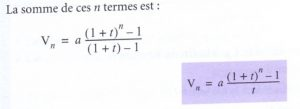formula end payment period has Vn = * (((1+t) power n) – 1) / t

formula payment period is Vn = a * ((((1+t) power n) – 1) / t) (1 + t)

payment end of period

VN = a * ((1+t) power n-1) / ((1+t)-1)

VN = a * ((1+t) power n-1) / t

Information necessary for the application of the formula:

• n = number of constant amounts paid;

• t = i, interest rate corresponding to the period;

• a = amount paid at the end of each period.

Beware this is the formula of the SUMS of the geometric sequence differently than before or it was to know the amount n of a suite.

example 1

end of period payments

This is for example 1000 euros paid to 10% for 3 years at end of period

1000 end first period they give so 2 years interest 10001,11,1=1210

1000 end second period so they give 1 year interest 1000 * 1, 1 = 1100

1000 end third period they give so 0an interests

1210 1100 + 1000 plus 3310

formula 1000 * (((1+10%) power 3) – 1) / 10% = 3310

At the beginning of period

1000 paid beginning to end first period they give so 3 years interest 10001,11,1*1,1=1331

1000 paid beginning to end first period they give so 2 years interest 10001,11,1=1210

1000 paid beginning to end first period so they give 1 year interest 1000 * 1, 1 = 1100

end period 1000 formula * (((1+10%) power 3) – 1) / 10% = 3310

What is wrong

as 1100 + 1210 1331 = 3641

The right formula is 1000 * ((((1+10%) power 3) – 1) / 10%) *(1+10%) = 3641

or 1000 (1 + 10 %)((((1+10%) 3 power) – 1) / 10%) = 3641

example 2

50000 euros are paid all the end of year for 12 years, 10% interest rate

Attention as there are payment at year end, there are 11 period and not 12 as pour 50000 late 12 th, but it n ' is not accruing interest.

V12 = 50000 (1 + 10%) power 11

50000 (1 + 10%) power 10

50000 (1 + 10%) power 9

50000 (1 + 10%) power 8

50000 (1 + 10%) power 7

50000 (1 + 10%) power 6

50000 (1 + 10%) power 5

50000 (1 + 10%) power 4

50000 (1 + 10%) power 3

50000 (1 + 10%) power 2

50000 (1 + 10%) power 1

50000 (1 + 10%) power 0, just 50000

regardless of the natural number n non-zero, 0n = 0 and 1n = 1 told 0 power n

so

V12 = 50000 (1 + 10%) power 11

50000 (1 + 10%) power 10

50000 (1 + 10%) power 9

50000 (1 + 10%) power 8

50000 (1 + 10%) power 7

50000 (1 + 10%) power 6

50000 (1 + 10%) power 5

50000 (1 + 10%) power 4

50000 (1 + 10%) power 3

50000 (1 + 10%) power 2

• 50000 (1 + 10%)
• 50000

Beware this is the formula of the SUMS of the geometric sequence

V12 = 50000 * (((1+10%) puissance12) – 1) / 0, 1 =

# Refresh

## Refresh

Discounting is calculating the value to our day a sum or multiple future sums.

That answers the question what is the current value of X euros x years

Discounting is calculating the value to our day a sum or multiple future sums while the CAP allows to know what will be in the future a sum of our days.

Discounting allows a number of things:

• Know what is currently worth a euro in the future. Indeed, discounting allows to predict the value of a euro in the future, regardless of the period although this period is often of a year.
• Find out what will be equal amounts expected in the future.
• To compare projects that give several irregular payments. When considering an investment project, it is necessary to predict the annual results expected over several years. Then meet in the presence of a suite of money to bring back to one, to compare either the amount invested, the expected amount of other investment projects. These are, related to different dates, will be updated to the same date (in general, if we start to today's date, the date 0).
• To support the applications of laws. Today, accounting standards require that the end of each fiscal year of a business is subject to a discount in order to evaluate certain positions of the company. The CCP tells us that, at the end of each fiscal year, the company undertake a refresh to evaluate certain positions of the balance sheet. When there is an impairment of assets property, its 'real' (or current) value must be determined in order to measure the suffered depreciation. We're holding the higher of the following two values:-market value: amount obtainable from the sale of assets;-use value: amount updated of future economic benefits expected from the good. Discount calculations are essential in finance. We'll eventually find them on several occasions.
• Get a rate of return. A rate of return to income we hope to get to a project. A project is a series of income. Judge the project and determine whether it is satisfactory compared to the cost of money (credit or dividends to be paid) often requires to calculate the expected rate of return on the basis of projected revenues compared to a reference (such as the cost of money) rate or the rate of return offered by other investments.

### Closely related to the present value discount rate

The current value of a capital will tend to be reduced depending on the rate and duration of discounting, it's normal because we're in the opposite direction of compound interest.

## The formulas

In order to simplify the thing, it is important that the refresh comes back to do the inverse calculation of capitalization or remove interests to the sum that interests us.

### Refresh a single sum

This amounts to calculate the value of a capital that will be received in a number of periods of our days taking to party that the capital is to compound interest.

Example

Either a capital of € 2,000 to perceive in 2 years.

What is the value of this capital today (at the time 0), if we use a discount rate of 5%?

We can say that the value today (C0) placed at 5% should give 2,000 after 2 years, where:

C0 (1.05) 2 = 2 000-online C0 = 2000 /(1,05puissance2) = 2 000 (1.05) power 2 = 1814,058957

so formula of the capitalisation1814, 058957 * (1.05) power 2 = 2000

It is equivalent to have now 1814 or 2000 euros in two years.

It is like using K as capital, as discount rates and N t for the year (or the period that correcpond), we get a calculation such as:

K (for by examples 0 periods) is equal to K factor of 1 + t the whole period to the negative exponent.

or

t designating the discount rate, the present value of a single C, n periods before capital, is given by the formula:

C0 = C (1 + 1) power n

### Update of a series of constant amounts paid at end of period

A constant sum is paid at the end of each period (if it is years, the first sum is paid at the end of the first year).

So we have

V0 = a(1+t) power-1 + power-2 a(1+t) + a(1+t) power-3 + power-n a(1+t)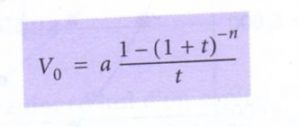V0 = a (1-(1 + t) power-n) / t

If payments are made at the beginning of period the formula becomes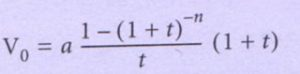V0 = a ((1-(1 + t) power-n) / t) (1 + t)

We can say that the amount of the loan is equal to the value of annuities, updated at the rate of the loan.

annuities constant = amount of the loan * interest rate / (1-(1+taux) power-number year)

example:

Consider a loan of €100,000 at the rate of 5% refundable by constant instalments of end of period 6 years.

QUESTIONS

1. Calculate the amount of the annuity constant. as a reminder, the formula is

annuities constant = amount of the loan * interest rate / (1-(1+taux) power-number year)

1. Calculate the value of the debt in 4 years before the deadline (the end of the second year).

SOLUTION

Constant annuity:

100 000 * 0.05 = 19701 /(1-1,05puissance-6), €74

The company will pay €19 701,74 to the Bank at the end of every year for 6 years.

Amount of the debt to the end of the second year (after the release of the 2nd annual fee): on this date, it remains still 4 annuities to be paid. The amount of the loan outstanding can be determined by discounting these 4 annual fees at the rate of the loan

V0 = a (1-(1 + t) power-n) / t

Vo = 19701, 74 * (1-1, 05puissance-4) / 0, 05 = 69861, 39

At the end of the second year, the value of the debt is 861,39 €69 (amount to be paid if the company decided to repay on that date).

### Updating of a sum over a fixed or infinite period

It may happen that the flow of a title are so long that the title is ours: in which case the end of period dividend is said constant. It is then a pension, it's really very interesting financially speaking, especially if the annuity follows inflation.

The formulaIf n tends to infinity (see the limits, high school courses). Then (1 + t) power-n closer (they say it tends) to 0 so if n is infinite formula can be simplified by

V0 = income/t

example I buy an action that gives €10 indefinitely, the rate is 5%, what is the value of this action?

V0 = 10/0.05 = 200

# The rate of actuarial return

There may be times that you have all the knowledge of the operation except one: the rate of this operation. If this is the case, just to vary the unknown factor of the previous theme.

The actuarial t of an investment rate of return is the gain obtained during the term of this investment expressed in the form of an annual rate.

## The vocabulary point

• This stranger is the rate of return the concerned investment actuarial. For this rate, there is equivalence between the sum placed (or invested) and the present value of the payments received in return. Clearly, it is the total of the gains made during the placement will be given in the form of a rate.

## Formulas

### A single value

Example: You pay 10,000 euro and we promise 11000 in 3 years, what is the rate?

You can go to

10000 = 11000(1+t) power-3

or 10000 *(1+t) puissance3 = 11000

10000 = 11000(1+t) power-3 is equivalent to 10000/11000 =(1+t) puissance3 equals t =(11000/10000) (1/3 power) – 1 = 3, 23%

t =(somme final/somme initiale) (power of 1/n)-1

### Cases with multiple flow

You're going to have to try either excel or by trial and error…

### Amount to be paid to achieve a rate of return desired known

• Let's assume we know the payments that will be obtained as well as the rate of return that we would, there more to find the basic amount that must be paid

Clear: that which is paid at the beginning and what will be received later in compensation to obtain the rate of yield of the operation

Where the rate of return is previously imposed, then the only likely to vary point is the basic amount.

If you do a refresh of the amounts that are expected, we can find the amount necessary to place.

Thanks to the update, can be found, if you know the other elements:

• the value of a sum or sums to its base date suite;
• the rate of return of an investment;
• either the amount of the fixed cash payments to derive a rate of return.
•

• Two rates are to be proportionate, must be equal to the ratio of the corresponding periods the relationship between them.
• Example

• Both rates are equivalent, then when used on a same capital during the same period, their results must be equal
• Example

• Banks use rates that are proportional to annual rates or rates for a given period when payments are made before the end of this period. This amounts therefore to do use an equivalent annual rate which will be stronger than it officially announced.## Veux-tu  commencer à construire ton patrimoine ?

Profites pour commencer à t'enrichir et exploites le système grâce à ce premier placement jusqu'à 10%
Je veux moi aussi gagner
* vous souscrivez à la newsletter richesse et finance et vous serez redirigé vers l'opportunité d'investissement du moment (lendix)## Recevez nos petits secrets pour devenir riche.

Profitez-en pour recevoir gratuitement par mails tout ce que vous avez besoin:
-les bons plans, les informations exclusives, les meilleurs conseils...
J'en profite